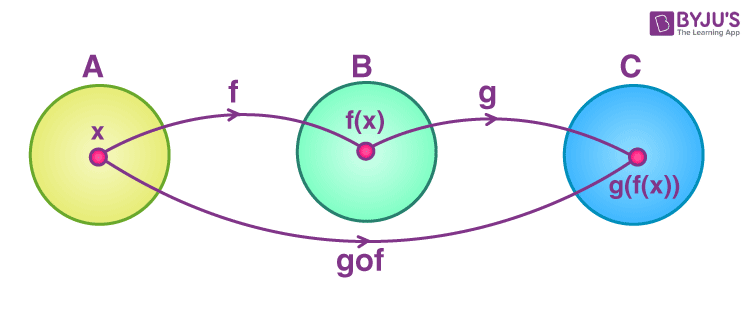# Composition of Functions

In Maths, the composition of a function is an operation where two functions say f and g generate a new function say h in such a way that h(x) = g(f(x)). It means here function g is applied to the function of x. So, basically, a function is applied to the result of another function.

Let’s have a look at the definition of a composite function.

## Composite Functions Definition

Let f : A → B and g : B → C be two functions. Then the composition of f and g, denoted by g ∘ f, is defined as the function g ∘ f : A → C given by g ∘ f (x) = g(f (x)), ∀ x ∈ A.

The below figure shows the representation of composite functions.The order of function is an important thing while dealing with the composition of functions since (f ∘ g) (x) is not equal to (g ∘ f) (x).

The relation and function is an important concept of Class 11 and 12. See below the function composition symbol and domain with example.

Symbol: It is also denoted as (g∘f)(x), where ∘ is a small circle symbol. We cannot replace ∘ with a dot (.), because it will show as the product of two functions, such as (g.f)(x).

Domain: f(g(x)) is read as f of g of x. In the composition of (f o g) (x) the domain of function f becomes g(x). The domain is a set of all values which go into the function.

Example: If f(x) = 3x+1 and g(x) = x2 , then f of g of x, f(g(x)) = f(x2) = 3x2+1.

If we reverse the function operation, such as f of f of x, g(f(x)) = g(3x+1) = (3x+1)2

## Properties of Function Compositions

Associative Property: As per the associative property of function composition, if there are three functions f, g and h, then they are said to be associative if and only if;

f ∘ (g ∘ h) = (f ∘ g) ∘ h

Commutative Property: Two functions f and g are said to be commute with each other, if and only if;

g ∘ f = f ∘ g

Few more properties are:

• The function composition of one-to-one function is always one to one.
• The function composition of two onto function is always onto
• The inverse of the composition of two functions f and g is equal to the composition of the inverse of both the functions, such as (f ∘ g)-1 = ( g-1 ∘ f-1).

### How to Solve Composite Functions

In maths, solving a composite function signifies getting the composition of two functions. A small circle (∘) is used to denote the composition of a function.

Go through the below-given steps to understand how to solve the given composite function.

Step 1: First write the given composition in a different way.

Consider f(x) = x2 and g(x) = 3x

Now,

(f ∘ g) (x) can be written as f[g(x)].

Step 2: Substitute the variable x that is there in the outside function with the inside function by taking the individual functions as a reference.

That means,

(f ∘ g)(x) = f(3x) {since g(x) = 3x}

Step 3: Finally, simplify the obtained function.

(f ∘ g)(x) = f(3x) = (3x)2 {since f(x) = x2}

= 9x2

### Function Composition With Itself

It is possible to compose a function with itself. Suppose f is a function, then the composition of function f with itself will be

(f∘f)(x) = f(f(x))

Let us understand this with an example:

Example: If f(x) = 3x2, then find (f∘f)(x).

Solution: Given: f(x) = 3x2

(f∘f)(x) = f(f(x))

= f (3x2)

= 3(3x)2

= 3.9x2

= 27x2

### Example of Composition of Functions

Q.1: If f (x) = 2x and g(x) = x+1, then find (f∘g)(x) if x = 1.

Solution: Given, f(x) = 2x

g(x) = x+ 1

Therefore, the composition of f from g will be;

(f∘g)(x) = f(g(x)) = f(x+1) = 2(x+1)

Now putting the value of x = 1

f(g(1)) = 2(1+1) = 2 (2) = 4

Q.2: If f(x) = 2x +1 and g(x) = -x2, then find (g∘f)(x) for x = 2.

Solution: Given,

f(x) = 2x+1

g(x) = -x2

To find: g(f(x))

g(f(x)) = g(2x+1) = -(2x+1)2

Now put x =2 to get;

g(f(2)) = -(2.2+1)2

= -(4+1)2

=-(5)2

=-25

Q.3: If there are three functions, such as f(x) = x, g(x) = 2x and h(x) = 3x. Then find the composition of these functions such as [f ∘ (g ∘ h)] (x) for x = -1.

Solution: Given,

f(x) = x

g(x) = 2x

h(x) = 3x

To find: [f ∘ (g ∘ h)] (x)

[f ∘ (g ∘ h)] (x) = f ∘ (g(h(x)))

= f ∘ g(3x)

= f(2(3x))

= f(6x)

= 6x

If x = -1, then;

[f ∘ (g ∘ h)] (-1) = 6(-1) = -6

Test your knowledge on Composition Of Functions